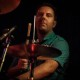adc - 4 years ago 90
Ruby Question

# Ruby only checks for first element of a boolean expression?

Here's my code:

``````array = ["b", "c", "a", "e", "d", "g", "i", "f"]
array.each_index do |letter|
if array[letter] == ("a" || "e" || "i" || "o" || "u")
puts "found #{array[letter]}"
end
end
``````

I'd expect it to return this:

``````found a
found e
found i
``````

but instead I only get

``````found a
``````

What's more, if I change the order of the elements within () on line 3, e.g. like this

``````if array[letter] == ("e" || "a" || "i" || "o" || "u")
``````

the code returns
`found e`
instead of
`found a`
.

I think I understand the problem—Ruby is only checking
`array`
for the first element within (). But can anyone explain why this is? I'd expect it to check for all of them.J&#246;rg W Mittag
Answer Source

`x || y` is `x` if `x` is truthy, `y` otherwise. `"a"` is truthy (everything except `nil` and `false` is truthy, those two and only those two are falsey), therefore `"a" || whatever_it_doesnt_matter` is always `"a"`.

So,

``````if array[letter] == ("a" || "e" || "i" || "o" || "u")
``````

is equivalent to

``````if array[letter] == ("a" || ("e" || ("i" || ("o" || "u"))))
``````

which evaluates to

``````if array[letter] == ("a" || ("i" || ("o" || "u")))
``````

which evaluates to

``````if array[letter] == ("a" || ("o" || "u"))
``````

which evaluates to

``````if array[letter] == ("a" || "u")
``````

which evaluates to

``````if array[letter] == "a"
``````
Recommended from our users: Dynamic Network Monitoring from WhatsUp Gold from IPSwitch. Free Download